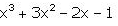Courses

# Can you explain the answer of this question below:What is the coefficient of x inA:-1B:-2C:3D:1The answer is b. Related Test: Test: Introduction To Polynomials

## Class 9 QuestionArt And Craft With Me 2 weeks ago
Option BMehul Agrawal 2 weeks ago
-2 is the answer because before ' x ' only -2 comesPriya Choudhary Jun 11, 2018
The answer will be -2 because coffecient of any variable is the first number before it.hence -2 is written before x .so it is the coffecient and if the question would be -x then its coefficient would be -1.Hope u understood my answerSadya Naaz Jun 28, 2018
X= -2 x xChinu Srivastav May 14, 2021
-2Harsh Sinha May 17, 2021
-2 is the answer
please follow me to get correct answerAlyssa Suri 2 weeks ago
Coefficient of x = -2

HoPe It HeLpS!
ThAnKs!Nipun Thakare May 07, 2021
B】-2Monika Singh Jul 16, 2020
BJatya Arjilli May 17, 2021
Yes because infront of the variable 'x' the constant is '-2' okay.
hope this answer helps you out

This discussion on Can you explain the answer of this question below:What is the coefficient of x in A:-1B:-2C:3D:1The answer is b. is done on EduRev Study Group by Class 9 Students. The Questions and Answers of Can you explain the answer of this question below:What is the coefficient of x in A:-1B:-2C:3D:1The answer is b. are solved by group of students and teacher of Class 9, which is also the largest student community of Class 9. If the answer is not available please wait for a while and a community member will probably answer this soon. You can study other questions, MCQs, videos and tests for Class 9 on EduRev and even discuss your questions like Can you explain the answer of this question below:What is the coefficient of x in A:-1B:-2C:3D:1The answer is b. over here on EduRev! Apart from being the largest Class 9 community, EduRev has the largest solved Question bank for Class 9.